Correlation tightness indicators for multivariate correlation-regression model

The tightness of the relationship between the studied indicators with multiple correlation is determined on the basis of various coefficients. In order for the regression equation to adequately reflect (approximate) the real simulated socio-economic processes or phenomena, the conditions and requirements of multiple correlation-regression analysis must be met.

Correlation - regression analysis : an analytical expression of the equation (rectilinear, curvilinear) regression for a multifactorial correlation and regression model. Definition of parameters and their interpretation.

The tightness of the relationship between them is measured by the ratio of factor variance to the total variance of the resultant attribute, called the determination index. The determination index characterizes the share of variation of the productive trait under the influence of the factor trait in the overall variability of the productive trait. If there is a correlation between the signs, then as it intensifies, i.e. increasing the tightness of the relationship between productive and factor signs, the determination index increases, and decreases as it weakens. Thus, the determination index characterizes the tightness of the relationship, the proximity of the correlation to the functional.

The square root of the determination index is the correlation index or theoretical correlation ratio . The correlation index, or theoretical correlation ratio, characterizes the tightness of the relationship for any form of dependence. Residual dispersion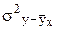necessary to select the best function that aligns (approximates) the empirical regression line to the greatest extent. The approximating function is selected by the minimum residual variance s 2 ost = S (y t -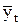) 2 / n or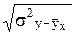.

A particular case of the correlation index is a linear correlation coefficient r , which is used to assess the tightness of the relationship with a linear relationship. The correlation coefficient takes values ​​from -1 to +1, showing not only crowdedness, but also the direction of communication. The “+” sign indicates a direct relationship between productive and factorial signs, the “-” sign indicates an inverse relationship between them. If r = 0, then there is no connection between the signs. The closer r is to unity, the closer is the relationship between the considered features.

In the linear form of communication, the parameter of the equation of the straight line is the regression coefficient a 1 and the correlation coefficient r are interconnected as follows:

and 1 = rs y / s x . In a straightforward relationship, the linear correlation coefficient is identical to the correlation index, they are numerically equal to: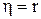.

The linear correlation coefficient r is used to assess the tightness of the connection with a linear relationship: direct equation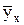= a 0 + a 1 xTo simplify the calculations of the linear correlation coefficient, use the transformed formula: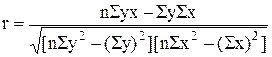.

The nature of the relationship is determined by the value of the correlation coefficient:

 r correlation coefficient nature of communication r = 0 before0.3 practically absent 0

The significance of the linear correlation coefficient is determined by t - student criterion. The calculated value of t calculation is determined, which is compared with the tabular value of t crit . The linear correlation coefficient is considered significant if the relation is observed: t calc > t crit .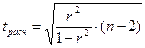at nfor n <50.

t crit is determined by the table "The value of t - student criterion at a significance level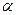0.10, 0.05, 0.01 and degrees of freedom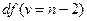.

The task of multivariate correlation - regression analysis is, firstly, to study a number of factors affecting the indicator under study and the selection of the most significant; secondly, in determining the degree of influence of each factor on an effective sign by constructing a model - the multiple regression equation, which allows you to establish in which direction and by what value the effective indicator will change when each factor in the model changes; thirdly, in the quantitative assessment of the tightness of the relationship between the effective attribute and factor.

Mathematically, the problem is to find an analytical expression of a function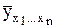= f (x 1 , x 2 , x 3 , ..., x n ), which in the best way reflects the connection of factor attributes with the effective one. The results of theoretical analysis and the possibility of their application to practice depend on the correct choice of the regression function, therefore, the form of communication should in the best way correspond to real-life relationships between the resultant attribute and the factor ones. The difficulty in choosing a function is that an effective attribute with different factors can be in various forms of communication - straightforward and curved. The empirical justification of the type of function using graphs of paired relationships is practically unsuitable for multiple correlation and regression.

The choice of the form of the multiple regression equation is based on a theoretical analysis of the phenomenon under study. If the analysis of the relationship between the productive and factor attributes does not allow us to dwell on any form of communication, then we sort through various functions and choose the optimal one from the point of view of proximity of the empirical values ​​of the resultant attribute to equal ones, but this is associated with the considerable complexity of calculating the parameters of various equations. If there is special software that implements an algorithm for enumerating various multiple regression equations on a PC, several models are obtained, the best one is selected by statistical verification of the parameters of the equation based on the Student t- test and Fisher F-test .

based on the use of five types of models :

linear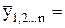a 0 + a 1 x 1 + a 2 x 2 + ... + a n x n ;

exponential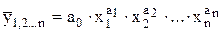;

indicative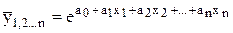;

parabolic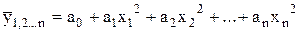hyperbolic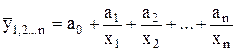Most often stop on linear models. This is due to the fact that, firstly, the parameters of linear equations are easily interpreted, the models themselves are simple and convenient for economic analysis, and secondly, if desired, any function can be reduced to a linear form by logarithming or replacing variables.

In the equation of multiple regression in linear form, the parameters a 1 , a 2 , a 3 , ..., and n are the regression coefficients, show the degree of influence of the corresponding factors on the effective sign when the remaining factors are fixed at an average level, i.e. how much will y change with an increase in the corresponding factor by 1 point in its unit of change; the parameter a 0 is a free term; it has no economic sense.

The parameters of the multiple regression equation , like the pair one, are calculated by the least squares method based on the solution of a system of normal equations. Since the regression coefficients are not comparable with each other (factors have different units of measurement), it is impossible to compare the strength of the influence of each of the factors included in the model on the effective sign based on the regression coefficients. To assess the comparative strength of the influence of factors, the partial elasticity coefficients and b-coefficients are calculated.

The partial coefficient of elasticity shows how many percent on average the effective indicator will change when the factor changes by 1% and the other factors are fixed and are calculated separately for each factor: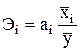, where a i is the regression coefficient at the i-th factor;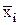- the average value of the i-th factor;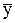- the average value of the effective indicator.

The b-coefficient shows how much of the standard deviation the resultant attribute changes when the corresponding factor changes by the value of its mean square deviation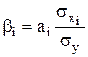, where s xi , s y are the mean square deviations of the i-th factor and the resultant attribute.

Due to the fact that economic phenomena are influenced by numerous and complex reasons, significant, systematically acting factors should be included in the multiple regression equation when eliminating the influence of other factors. The most important factors are selected on the basis of the analysis of the tightness and materiality of the relationship between the factors and the effective indicator. Moreover, the condition for including factors in the model is the absence of a very close correlation between them, close to functional. The presence between the two factors of a very close linear relationship (the linear correlation coefficient r exceeds the absolute value of 0.85) is called collinearity , and between several factors - multicollinearity .

The reasons for the occurrence of multicollinearity between features are, firstly, that the features being analyzed characterize the same side of the phenomenon or process (for example, the authorized capital and the number of employees characterize the size of the enterprise) and it is not advisable to include them in the model at the same time; secondly, factor signs are components of each other, duplicate each other, or their total value gives a constant value (for example, energy ratio and capital ratio, the proportion of borrowed and own funds). If multicollinear factors are included in the model, then the regression equation will inadequately reflect real economic relationships, the model parameters will be distorted (overestimated), the meaning will be changed and the economic interpretation of regression and correlation coefficients will be difficult.

Therefore, when constructing a model, one of the collinear factors is excluded on the basis of a qualitative and logical analysis, or the initial factor signs are transformed into new, enlarged ones. The quality and adequacy of the model to the real socio-economic phenomenon and process is determined by the optimality of the number of factor attributes: the more factors are included, the model describes the phenomenon and process better, but such a model is difficult to implement; with a small number of factors, the model is not adequate enough.

The problem of selecting factor features and reducing the dimension of the multiple correlation model is solved on the basis of heuristic and multidimensional analysis methods. The heuristic methods of analysis include the method of expert assessments, based on intuitive-logical prerequisites and a meaningful and qualitative analysis of nonparametric indicators of communication tightness: rank correlation coefficients, concordance. The most commonly used method is stepwise regression , which consists in sequentially including factors in the model and assessing their significance.

When introducing the factor, it is determined how much the sum of the squared residuals decreases and the value of the multiple correlation coefficient R increases . If, when the factor x k is included in the model, the value of R increases and the regression coefficient a k does not change or does not change significantly, then this factor is significant and its inclusion in the model necessary.

· The totality of the studied indicators should be homogeneous according to the conditions for the formation of effective and factor signs (distinguished observations should be excluded from the totality);

· The effective sign must obey the normal distribution law, factor - should be close to the normal distribution. If the volume of the population is large enough (n> 50), then the normality of the distribution can be confirmed by calculating and analyzing the criteria of Pearson, Yastremsky, Kolmogorov, Boyarsky, etc .;

· The simulated phenomenon or process is described quantitatively (the parameters must be digitally expressed) by one or more equations of cause and effect relationships. It is advisable to describe cause-effect relationships as linear or close to linear form dependencies;

· The constancy of the territorial and temporal structure of the studied population, the absence of quantitative restrictions on the parameters of the model;

· Sufficiency of aggregate units : their number should be several times greater than the number of factors included in the model. Each factor should have at least 5–6 observations, i.e. the number of factor signs should be 5-6 times less than the volume of the studied population.

The main stages of the correlation and regression analysis are:

· Preliminary theoretical analysis of the essence of the phenomenon, allowing to establish causal relationships between the signs, choose the most important factors, solve the problem of measuring the effective and factor signs;

· Preparation of baseline information , including questions of the adequacy of the units of observation, the uniformity of the totality of the studied features and the proximity of their distribution to normal;

· The choice of the form of communication between the effective attribute and factors based on enumeration of several analytical functions;

· The study of the tightness of the relationship between the productive trait and factors, as well as between factors based on the construction of a matrix of paired linear correlation coefficients and screening of multicollinear factors;

· Selection of significant (significant) factors included in a multivariate model - the multiple regression equation, based on relevant statistical methods;

· Calculating the parameters of the multiple regression equation and assessing the significance of the selected factors, correlation and regression coefficients using the t - Student and F - Fisher criteria;

· Analysis of the results.

The relationships between the signs are analyzed, as a rule, on the basis of sample observations, therefore, to verify that the obtained dependencies are regular, and not random, the significance (significance) of the correlation and regression indicators is evaluated.

Correlation - regression analysis is used to assess business plan indicators and standard levels of economic indicators, reflecting the efficiency of using production resources, identifying available production reserves, conducting a comparative analysis, assessing the potential of enterprises, and short-term forecasting of production development.

The multiple regression equation allows you to find the theoretical, possible value of the effective indicator for certain values ​​of factor attributes.

The parameters of the multiple regression equation are calculated by the least squares method based on the solution of a system of normal equations. For a linear regression equation with n factors, a system of (n + 1) normal equations is constructed: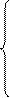a 0 n + a 1 Sx 1 + a 2 Sx 2 + ... + a n Sx n = Sy,

a 0 Sx 1 + a 1 Sx 2 1 + a 2 Sx 1 x 2 + ... + a n Sx 1 x n = Syx 1 ,

:

a 0 Sx n + a 1 Sx 1 x n + a 2 Sx 2 x n + ... + a n Sx 2 n = Syx n .

The tightness of the relationship between the studied indicators with multiple correlation is determined on the basis of various coefficients.

The paired correlation coefficients r measure the tightness of the linear relationship between factors and between the productive attribute and each of the considered factors without taking into account their interaction with other factors

Partial correlation coefficients characterize the degree of influence of factors on a productive attribute, provided that other factors are fixed at a constant level. Depending on the number of factors whose influence is excluded, the partial correlation coefficients can be of the first order (when excluding the influence of one factor), second order (when excluding the influence of two factors), etc.

The partial correlation coefficient of the first order between y and x 1 with the exclusion of the influence of x 2 in a two-factor model is calculated by the formula:,

where r yx 1 , r yx 2 , r x1x2 are paired correlation coefficients between the corresponding features.

The aggregate coefficient of multiple correlation R estimates the tightness of the relationship between the effective attribute and all factors. This is the main indicator of linear multiple correlation. For a two-factor model, the total multiple correlation coefficient is calculated by the formula:. The aggregate correlation coefficient R varies from 0 to 1. The smaller the empirical values ​​of the resultant attribute differ from those aligned along the line of multiple regression, the closer the correlation between the studied parameters is closer and the aggregate coefficient of multiple correlation is closer to unity.

The aggregate coefficient of multiple determination , equal to R 2 , shows which part of the variation of the effective trait is due to the influence of factors included in the model.

The aggregate index of multiple correlation characterizes the tightness of the relationship between the effective attribute and all factors with a curvilinear dependence: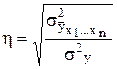=where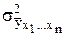- variance of the effective attribute under the influence of factors included in the model;- residual variance of the effective attribute caused by the influence of factors not taken into account by the model. In the linear form of communication, the aggregate coefficient and the multiple correlation index are equal.

The significance of the multiple correlation coefficient R is determined by the F - Fisher test. The calculated value of F calculation is determined, which is compared with the table value of F crit . Коэффициент множественной корреляции считается значимым при соблюдении соотношения: F расч > F крит .or,